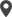Enable Dark Mode!By: Afra MP

# How to Add Inverse Function for Compute Fields in Odoo 16

## Technical Odoo 15

Odoo, a robust open-source business application suite, offers developers a wide array of tools and features to tailor and enrich the functionality of their ERP systems. Computed fields constitute a potent feature enabling automatic calculation and display values based on the values of other fields in a model. However, by default, Odoo does not provide a built-in way to define an inverse function for computed fields. In this blog, we will explore how you can implement an inverse function for compute fields in Odoo 16.

## What is a computed field?

A computed field is a field that is not stored directly in the database but is instead calculated on-the-fly based on the values of other fields or data. Computed fields allow you to perform calculations or manipulations on existing data within a model and present the derived result as if it were a regular field in the database. The function's name begins with _compute_function_name. The value of the compute field cannot be changed.

### Compute function

Computed fields in Odoo are fields that are dynamically calculated based on the values of other fields. They are defined using the @api.depends decorator in the model, which indicates the dependencies of the computed field.

For example, let's consider that we have a model and want to calculate the total amount based on the cost and quantity of each order line. We can define a computed field total_amount as follows:

`order_lines = fields.One2many('sale.order.line', 'order_id', string='Order Lines')    total_amount = fields.Float(string='Total Amount', compute='_compute_total_amount')    @api.depends('order_lines.price', 'order_lines.quantity')    def _compute_total_amount(self):        for order in self:            order.total_amount = sum(order.order_lines.mapped(lambda line: line.price * line.quantity))`
In this example, the total_amount computed field depends on the price and quantity fields of the order_lines one2many field. Whenever the values of these fields change, the _compute_total_amount function will be called to update the value of total_amount.

### Inverse Function

The inverse function is a method that is called when the value of a computed field is changed. The purpose of the inverse function is to update the values of the fields that the computed field depends on, so that the computed field still returns the correct value.A compute field value cannot be altered by the user. An inverse parameter can easily be used to remedy this issue. It will enable editing of a compute field.
In the previous example, suppose we wanted to include an inverse function to update each order line's quantity whenever the total_amount field was changed. We can do this as follows:
`def _inverse_total_amount(self):        for order in self:            if order.total_amount:                average_price = order.total_amount / len(order.order_lines)                for line in order.order_lines:                    line.quantity = average_price / line.price            else:                for line in order.order_lines:                    line.quantity = 0.0`
In this updated example, the total_amount field now has an inverse='_inverse_total_amount' argument. This suggests that whenever the total_amount field receives a new value, the _inverse_total_amount method should be invoked.
The average price is determined using the _inverse_total_amount method by dividing the total amount by the quantity of order lines. The quantity of each order line is then updated in accordance with the new average price.
By including this inverse function, the quantity of each order line will be changed automatically to preserve the updated total amount anytime the total_amount field is modified.
By implementing an inverse function for computed fields, you can add more powerful functionality to your Odoo models. This allows you to not only calculate values based on other fields but also update the necessary fields automatically when the computed field is modified.
In this blog, we explored how to add an inverse function for compute fields in Odoo 16. By understanding the concepts of computed fields and how to define an inverse function, you can leverage this feature to build more dynamic and automated models in Odoo.

If you need any assistance in odoo, we are online, please chat with us.

### Recent Posts

#### How the Reception Report Works in Odoo 17 Inventory AppCalicut

Cybrosys Technologies Pvt. Ltd.
Neospace, Kinfra Techno Park
Kakkancherry, Calicut
Kerala, India - 673635London

Cybrosys Limited
Alpha House,
100 Borough High Street, London,
SE1 1LB, United KingdomKochi

Cybrosys Technologies Pvt. Ltd.
1st Floor, Thapasya Building,
Kochi, India - 682030.Bangalore

Cybrosys Techno Solutions
The Estate, 8th Floor,